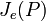# Join of elementary abelian subgroups of maximum order

Suppose$p$ is a prime number and$P$ is a finite p-group. The join of elementary abelian subgroups of maximum order in$P$, denoted$J_e(P)$, is defined as the join of all the elementary abelian subgroups that have maximum order among elementary abelian subgroups of$P$.